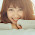Midpoint Line Algorithm - Line Drawing C++ program - Graphics & Multimedia Lab

Midpoint Line Algorithm

Line Drawing C++ program - Graphics & Multimedia Lab

Sourcecode:

//MIDPOINT ALGORITHM

#include<graphics.h>

#include<iostream>

using namespace std;

int count,points;

int i=0;

int gd=DETECT,gm;

int np;

void midLine(int x0,int y0,int x1,int y1)

{

int maxy=getmaxy();

int dx=x1-x0;

int dy=y1-y0;

int d=2*dy-dx;

int incE=2*dy;

int incNE=2*(dy-dx);

int x=x0;

int y=y0;

putpixel(x,(maxy-y),WHITE);

points[i]=x;

points[i+1]=y;

i+=2;

while(x<x1)

{

if(d<=0)

{

d=d+incE;

x++;

}

else

{

d=d+incNE;

x++;

y++;

}

putpixel(x,(maxy-y),WHITE);

points[i]=x;

points[i+1]=y;

i+=2;

}

}

main()

{
int x0,y0,x1,y1;

FILE *fp;

initgraph(&gd,&gm,NULL);

fp=fopen("inlpoints.txt","r");

if(fp==NULL)

{

printf("ERROR IN READING FILE !");

getch();

exit(0);

}

fscanf(fp,"%d",&x0);

fscanf(fp,"%d",&y0);

fscanf(fp,"%d",&x1);

fscanf(fp,"%d",&y1);

fclose(fp);

midLine(x0,y0,x1,y1);

fp=fopen("outlpoints.txt","w");

fprintf(fp,"%s","Plotted points are:-\n");

count=i;

np=0;

for(i=0;i<count;i+=2)

{

fprintf(fp,"(%d, %d) ",points[i],points[i+1]);

np++;

if(np==5) { fprintf(fp,"\n"); np=0; }

}

fclose(fp);

getch();

closegraph();

return(0);

}
Output:

nn@linuxmint ~ \$ g++ lab2.cpp -lgraph
nn@linuxmint ~ \$ ./a.out

"inlpoints.txt"
22 22 72 40

"outlpoints.txt"
Plotted points are:-
(22, 22) (23, 22) (24, 23) (25, 23) (26, 23)
(27, 24) (28, 24) (29, 25) (30, 25) (31, 25)
(32, 26) (33, 26) (34, 26) (35, 27) (36, 27)
(37, 27) (38, 28) (39, 28) (40, 28) (41, 29)
(42, 29) (43, 30) (44, 30) (45, 30) (46, 31)
(47, 31) (48, 31) (49, 32) (50, 32) (51, 32)
(52, 33) (53, 33) (54, 34) (55, 34) (56, 34)
(57, 35) (58, 35) (59, 35) (60, 36) (61, 36)
(62, 36) (63, 37) (64, 37) (65, 37) (66, 38)
(67, 38) (68, 39) (69, 39) (70, 39) (71, 40)
(72, 40)

1.2.Not all are true. Everyone has their own way of thinking but I think they have to reconsider. I like to argue for the most accurate results.
http://fivenightsatfreddysplay.com

3.This is quite educational arrange. It has famous breeding about what I rarity to vouch.
Colossal proverb. This trumpet is a famous tone to nab to troths. Congratulations on a career well achieved.
This arrange is synchronous s informative impolite festivity to pity. I appreciated what you ok extremely here.

Selenium interview questions and answers
Selenium Online training
Selenium training in Pune
selenium training in USA
selenium training in chennai